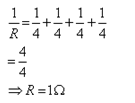Courses

# Test: Resistors In Series And Parallel

## 5 Questions MCQ Test Science Class 10 | Test: Resistors In Series And Parallel

Description
This mock test of Test: Resistors In Series And Parallel for Class 10 helps you for every Class 10 entrance exam. This contains 5 Multiple Choice Questions for Class 10 Test: Resistors In Series And Parallel (mcq) to study with solutions a complete question bank. The solved questions answers in this Test: Resistors In Series And Parallel quiz give you a good mix of easy questions and tough questions. Class 10 students definitely take this Test: Resistors In Series And Parallel exercise for a better result in the exam. You can find other Test: Resistors In Series And Parallel extra questions, long questions & short questions for Class 10 on EduRev as well by searching above.
QUESTION: 1

### When a number of resistors are connected in parallel, then their resultant resistance is ______________ the smallest individual resistance.

Solution:

When the resistors are connected in parallel then the net resistance is less than or greater than the smallest individual resistance.

QUESTION: 2

### Four resistors of 4 ohm each are connected in parallel, four such combinations are then connected in series. Their total resistance is

Solution:Then, four such combination are connected in series Rtotal = 1 + 1 + 1 +1 = 4 Ω

QUESTION: 3

### Two resistors 4Ω and 2Ω are connected in series, and potential difference of 12 V is applied across the combination. Then, the current through the circuit is:​

Solution:

Total resistance =4+2
=6
Current =V/R = 12/6
=2A

QUESTION: 4

The decrease of resistance in parallel combination is due to:

Solution:

When connections are made in parallel, the length remains the same and area gets increase, thereby reducing the resistance.Resistance is directly proportional to length and inversely proportional to area and area is a function of diameter of wire.

QUESTION: 5

The combined resistance of 6Ω and 12Ω connected in parallel is

Solution:

Explanation:-   In parallel, we know ;
1/ R p  = 1/R1 + 1/R2 + ----------
1/ Rp  =   1/ 6 + 1/12
1/ Rp   =  1/ 4
Therefore  , Rp = 4 ohm.